GRE Subject Test: Math : Classifying Functions

Example Questions

Example Question #1 : Classifying Functions

What kind of function is this: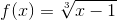?

Cube-Root Function

Square Function

Cube Function

Rational Function

Cube-Root Function

Explanation:

Step 1: Look at the equation..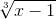. The cube-root outside of the function determines what the answer is..

The function is a cube-root function.

Note:

Square function,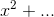Cube function,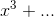Rational function,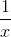(if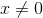)

Example Question #11 : Calculus

What kind of function is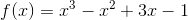Hyperbola

Line

Cubic

Parabola

Cubic

Explanation:

Step 1: To classify any function, find the degree of that function. The degree of a function is the highest exponent.

Step 2: Find degree of.

The degree is 3..

Step 3: Find what kind of function has degree of 3:

A cubic function has a degree of 3...

A square function has a degree of 2
A line has a degree of 1

Example Question #2 : Classifying Functions

What kind of function is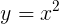Circle

Line

Parabola

Cubic

Parabola

Explanation:

Step 1: Define some basic graphs and their functions...

Line functions have an equation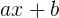, where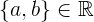Parabola (Quadratic functions) have an equation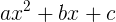or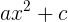or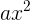, where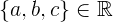Cubic functions have an equation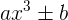, whereStep 2: Determine what type of function is given...

The equation given is a parabola...

Example Question #2 : Classifying Functions

What kind of function is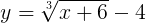?

Reciprocal Function

Absolute Value

Cubic Function

Cube-Root Function

Cube-Root Function

Explanation:

Quadratic functions have at least an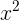term.
Absolute Functions have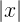.

Cube-Root functions have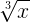The function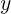is a cube-root function because it sharesAll GRE Subject Test: Math Resources